The PCAP badge covers the analysis of packet capture to retrieve information. It covers multiple protocols with an extensive focus on HTTP

Exercises

5

CPEs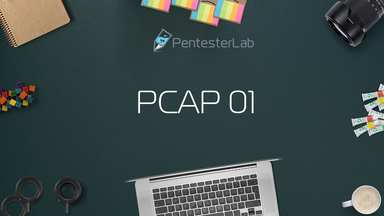PCAP 01

Difficulty: EASY
• 1 video
• Completed by 891 students
• Takes Less than an hour on average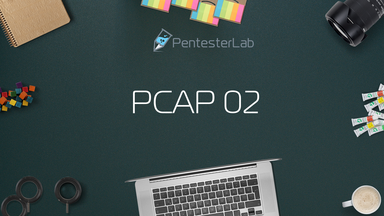PCAP 02

Difficulty: EASY
• 1 video
• Completed by 865 students
• Takes Less than an hour on average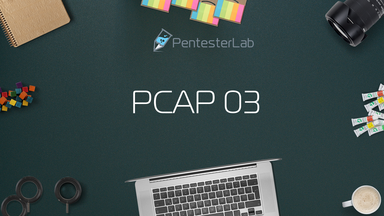PCAP 03

Difficulty: EASY
• 1 video
• Completed by 850 students
• Takes Less than an hour on average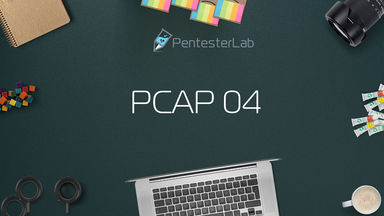PCAP 04

Difficulty: EASY
• 1 video
• Completed by 755 students
• Takes Less than an hour on average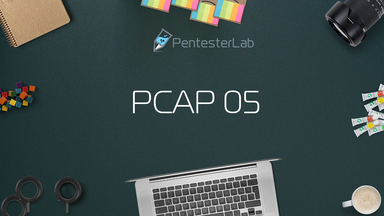PCAP 05

Difficulty: EASY
• 1 video
• Completed by 742 students
• Takes Less than an hour on average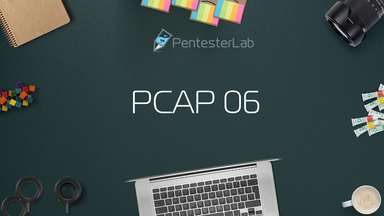PCAP 06

Difficulty: EASY
• 1 video
• Completed by 729 students
• Takes Less than an hour on average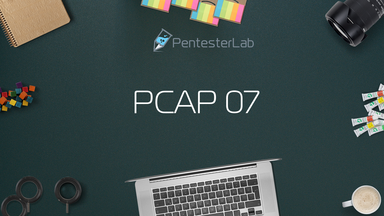PCAP 07

Difficulty: EASY
• 1 video
• Completed by 714 students
• Takes Less than an hour on average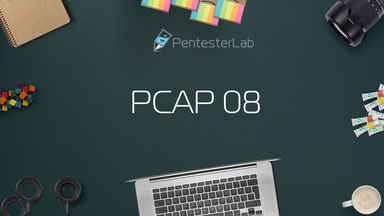PCAP 08

Difficulty: EASY
• 1 video
• Completed by 709 students
• Takes Less than an hour on average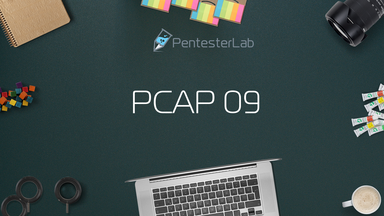PCAP 09

Difficulty: EASY
• 1 video
• Completed by 709 students
• Takes Less than an hour on average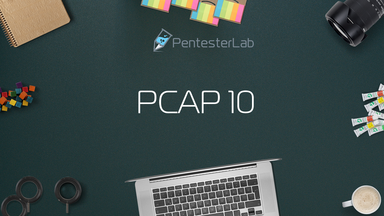PCAP 10

Difficulty: EASY
• 1 video
• Completed by 561 students
• Takes Less than an hour on average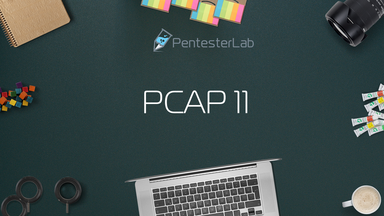PCAP 11

Difficulty: EASY
• 1 video
• Completed by 550 students
• Takes Less than an hour on average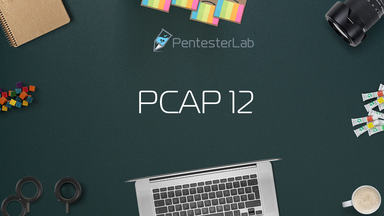PCAP 12

Difficulty: EASY
• 1 video
• Completed by 546 students
• Takes Less than an hour on average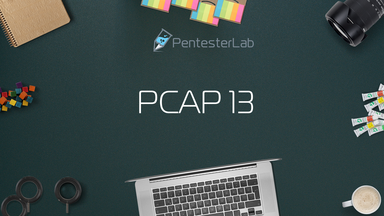PCAP 13

Difficulty: EASY
• 1 video
• Completed by 631 students
• Takes Less than an hour on average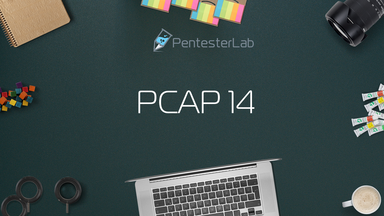PCAP 14

Difficulty: EASY
• 1 video
• Completed by 623 students
• Takes Less than an hour on average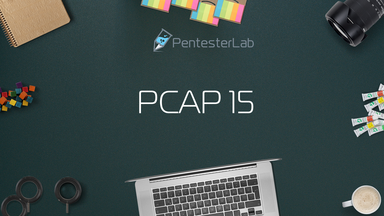PCAP 15

Difficulty: EASY
• 1 video
• Completed by 615 students
• Takes Less than an hour on averagePCAP 16

Difficulty: EASY
• 1 video
• Completed by 615 students
• Takes Less than an hour on averagePCAP 17

Difficulty: EASY
• 1 video
• Completed by 562 students
• Takes Less than an hour on average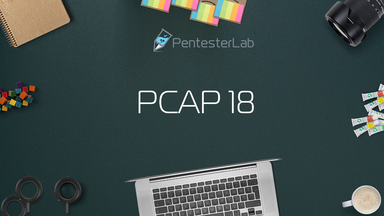PCAP 18

Difficulty: EASY
• 1 video
• Completed by 560 students
• Takes Less than an hour on average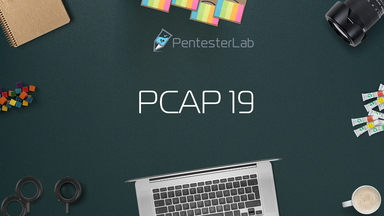PCAP 19

Difficulty: EASY
• 1 video
• Completed by 538 students
• Takes Less than an hour on average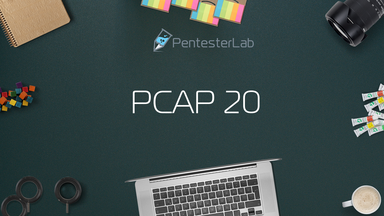PCAP 20

Difficulty: EASY
• 1 video
• Completed by 520 students
• Takes Less than an hour on average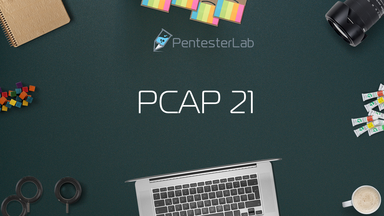PCAP 21

Difficulty: EASY
• 1 video
• Completed by 503 students
• Takes Less than an hour on average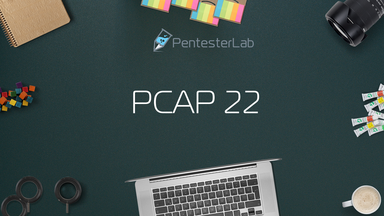PCAP 22

Difficulty: EASY
• 1 video
• Completed by 464 students
• Takes Less than an hour on average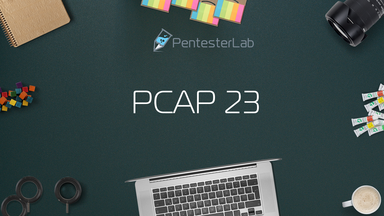PCAP 23

Difficulty: EASY
• 1 video
• Completed by 461 students
• Takes Less than an hour on average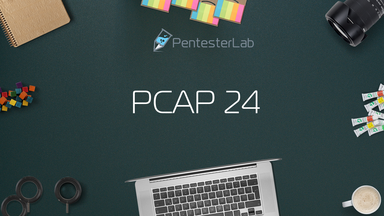PCAP 24

Difficulty: EASY
• 1 video
• Completed by 459 students
• Takes Less than an hour on average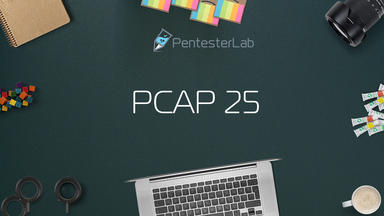PCAP 25

Difficulty: EASY
• 1 video
• Completed by 461 students
• Takes Less than an hour on average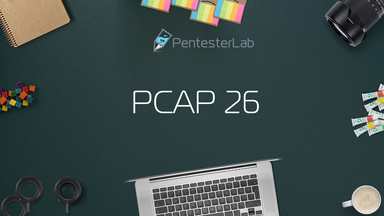PCAP 26

Difficulty: EASY
• 1 video
• Completed by 462 students
• Takes Less than an hour on average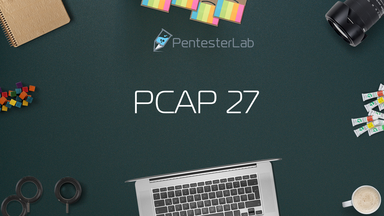PCAP 27

Difficulty: EASY
• 1 video
• Completed by 417 students
• Takes Less than an hour on average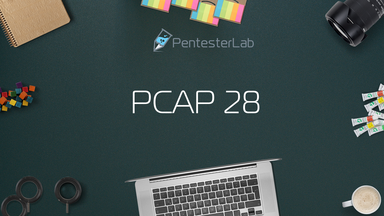PCAP 28

Difficulty: EASY
• 1 video
• Completed by 408 students
• Takes Less than an hour on average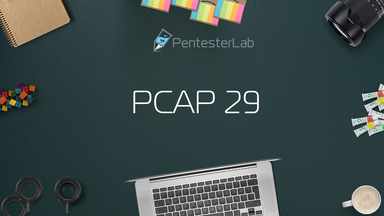PCAP 29

Difficulty: EASY
• 1 video
• Completed by 411 students
• Takes Less than an hour on average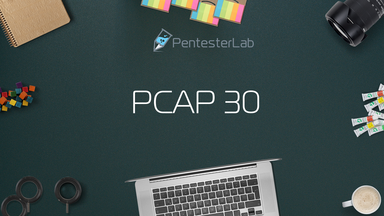PCAP 30

Difficulty: EASY
• 1 video
• Completed by 406 students
• Takes Less than an hour on average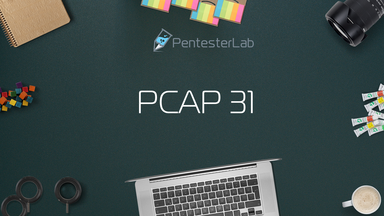PCAP 31

Difficulty: EASY
• 1 video
• Completed by 404 students
• Takes Less than an hour on average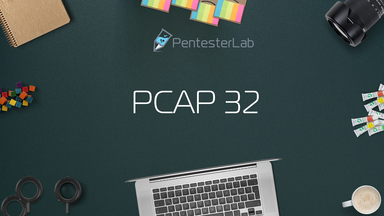PCAP 32

Difficulty: EASY
• 1 video
• Completed by 371 students
• Takes Less than an hour on average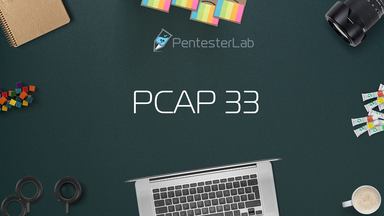PCAP 33

Difficulty: EASY
• 1 video
• Completed by 355 students
• Takes Less than an hour on average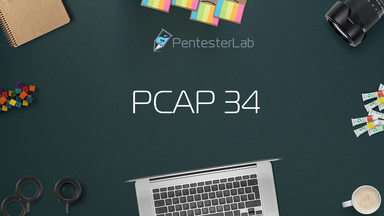PCAP 34

Difficulty: EASY
• 1 video
• Completed by 364 students
• Takes Less than an hour on average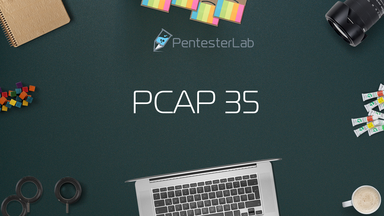PCAP 35

Difficulty: EASY
• 1 video
• Completed by 378 students
• Takes Less than an hour on average